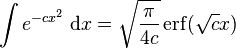# Unable to figure out integral

Eats Dirt

## Homework Statement

I have an integral in the form of
[/B]
$$\int_{0}^{\infty} e^{-(\frac{x-c}{b})^2}dx$$

and I can't seem to figure it out.

I feel like I need a special function (gamma?)

Last edited:

## Answers and Replies

Eats Dirt
Ooops I think I realized I need to do a change of variables...

Last edited:
Homework Helper
The LaTeX for infinity is \infty, which produces $\infty$.

To your problem: the square of that integral is $$\int_0^\infty \int_0^\infty e^{-(\frac{x-c}b)^2 - (\frac{y-c}b)^2}\,dx\,dy$$ and the substitution $$x - c = r \cos \theta \\ y - c = r \sin \theta$$ is called for.

Last edited:
•Eats Dirt
Homework Helper
Dearly Missed
Eats Dirt
would this work?

use change of variables

$$y=(\frac{x-c}{b})\\ dy=\frac{dx}{b}\\ substitute\\\\ \int_{0}^{\infty}e^{-ay^2}dy = \frac{1}{2}\sqrt{\frac{pi}{a}}$$

oops I did not make up for the change of variables in the lower limit of the integral

Last edited:
Staff Emeritus
Homework Helper
Almost. You need to fix the limits of integration.

Eats Dirt
$$\int_{-c/b}^{\infty}e^{-ay^2}dy =! \frac{1}{2}\sqrt{\frac{pi}{a}}$$

Now the Gaussian doesn't work in that form so I think I need the indefinite formLast edited:
Homework Helper
Dearly Missed
$$\int_{-c/b}^{\infty}e^{-ay^2}dy =! \frac{1}{2}\sqrt{\frac{pi}{a}}$$

Now the Gaussian doesn't work in that form so I think I need the indefinite formand I just evaluate this in my new limits?

You tell us.

Homework Helper
You could also just use some brute force and integration by parts:
let ##\alpha = \frac{x-c}{b}##
You will have ## \int_{\frac{-c}{b}}^\infty e^{-\alpha^2} d\alpha ##
by parts, ##u = e^{-\alpha^2}, \, du = -2\alpha u d\alpha ##
## v = \alpha, \, dv = d\alpha ##
## \int_{\frac{-c}{b}}^\infty e^{-\alpha^2}d\alpha = \left. uv \right|_{\frac{-c}{b}}^\infty + 2 \int_{\frac{-c}{b}}^\infty \alpha^2 e^{-\alpha^2} d\alpha ##
##\int_{\frac{-c}{b}}^\infty \alpha^2 e^{-\alpha^2} d\alpha ## can again be evaluated by parts...
substituting ##\beta = \alpha^2 ## gives ##-\int_{\frac{-c^2}{b^2}}^{-\infty} \beta e^{\beta} d\beta ##
Let ## u = \beta, \, du = d\beta ## and ## v=e^\beta, \, dv = e^\beta d\beta ## .
One more time, and keep track of your signs, do some algebra, and before you know it... Solved.
However, I think the suggesions above may prove to be more elegant.

Eats Dirt
You tell us.

Ok, now we use the error function,
$$erf(y)=\frac{2}{\sqrt{\pi}}\int_{0}^{y} e^-t^2 dt$$

After I evaluate the error function I should put in the proper integration limits (from the original function) of y? But doesn't this just lead us in circles now? to evaluate this function I need another Gaussian function in a form that does not run from 0 to infinity.

Homework Helper
Dearly Missed
Ok, now we use the error function,
$$erf(y)=\frac{2}{\sqrt{\pi}}\int_{0}^{y} e^-t^2 dt$$

After I evaluate the error function I should put in the proper integration limits (from the original function) of y? But doesn't this just lead us in circles now? to evaluate this function I need another Gaussian function in a form that does not run from 0 to infinity.

No, you are not running in circles. You are expressing an integral (the one you started with) in terms of a function "erf" (or equivalent). The latter is not an elementary function, but it is well-studied and has a standard definition.

The only real difference between a function like "erf" and functions like "sin", "cos", "exp" "log", etc, is that you saw these last ones early in your education and so you are more familiar with them and more practiced in their use. Also, you can probably find them on just about any decent hand-held calculator, while "erf" shows up later in your studies and needs a fancier calculator to implement it.

Homework Helper
The LaTeX for infinity is \infty, which produces $\infty$.

To your problem: the square of that integral is $$\int_0^\infty \int_0^\infty e^{-(\frac{x-c}b)^2 - (\frac{y-c}b)^2}\,dx\,dy$$ and the substitution $$x - c = r \cos \theta \\ y - c = r \sin \theta$$ is called for.

Actually the $\theta$ intergral here is not straightforward due to the nature of the boundary, so using the error function is easier.

Homework Helper
##\int_{-c/b}^\infty e^{-t^2} dt ## has the form of the complimentary error function ##1-erf(x)##, as described on the Wikipedia page linked above.
You can also read more at http://www.wolframalpha.com/input/?i=erfc(x) .
If you would prefer the expansion in terms of the given values without functional dependence on the erf, you can go through the somewhat messy integration I outlined above.

Eats Dirt
thank you all, I ended up getting the correct solution.